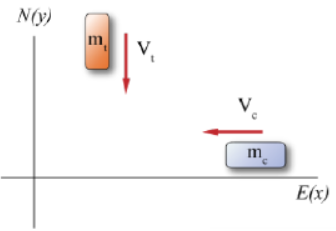# Problem: A car with mass mc = 1000 kg is traveling west through an intersection at a magnitude of velocity of vc  = 5.0 m/s when a truck of mass mt = 1500 kg traveling south at vt = 6.0 m/s fails to yield and collides with the car. The vehicles become stuck together and slide on the asphalt, which has a coefficient of friction with the rubber tires of μk = 0.5.How far, in meters, will the vehicles slide after the collision?

###### FREE Expert Solution

Friction force,

$\overline{){{\mathbit{f}}}_{{\mathbf{k}}}{\mathbf{=}}{{\mathbit{\mu }}}_{{\mathbf{k}}}{\mathbit{m}}{\mathbit{g}}}$

Work done,

$\overline{){\mathbit{W}}{\mathbf{=}}{{\mathbit{f}}}_{{\mathbf{k}}}{\mathbit{d}}}$

Kinetic energy,

$\overline{){\mathbf{K}}{\mathbf{E}}{\mathbf{=}}\frac{\mathbf{1}}{\mathbf{2}}{\mathbf{m}}{{\mathbf{v}}}^{{\mathbf{2}}}}$

Conservation of momentum,

$\overline{)\begin{array}{rcl}{\mathbf{m}}_{\mathbf{t}}{\mathbf{v}}_{\mathbf{0}\mathbf{t}}\mathbf{+}{\mathbf{m}}_{\mathbf{c}}{\mathbf{v}}_{\mathbf{0}\mathbf{c}}& \mathbf{=}& \mathbf{\left(}{\mathbf{m}}_{\mathbf{t}}\mathbf{+}{\mathbf{m}}_{\mathbf{c}}\mathbf{\right)}{\mathbf{v}}_{\mathbf{f}}\end{array}}$

Vector magnitude,

$\overline{)\begin{array}{rcl}\mathbf{|}\mathbf{v}\mathbf{|}& {\mathbf{=}}& \sqrt{{\mathbf{\left(}{\mathbf{v}}_{\mathbf{x}}\mathbf{\right)}}^{\mathbf{2}}\mathbf{+}{\mathbf{\left(}{\mathbf{v}}_{\mathbf{y}}\mathbf{\right)}}^{\mathbf{2}}}\end{array}}$

mc = 1000 kg

vc  = - 5.0 m/s î

m= 1500 kg

vt = - 6.0 m/s ju

μk = 0.5.###### Problem Details

A car with mass mc = 1000 kg is traveling west through an intersection at a magnitude of velocity of vc  = 5.0 m/s when a truck of mass mt = 1500 kg traveling south at vt = 6.0 m/s fails to yield and collides with the car. The vehicles become stuck together and slide on the asphalt, which has a coefficient of friction with the rubber tires of μk = 0.5.

How far, in meters, will the vehicles slide after the collision?